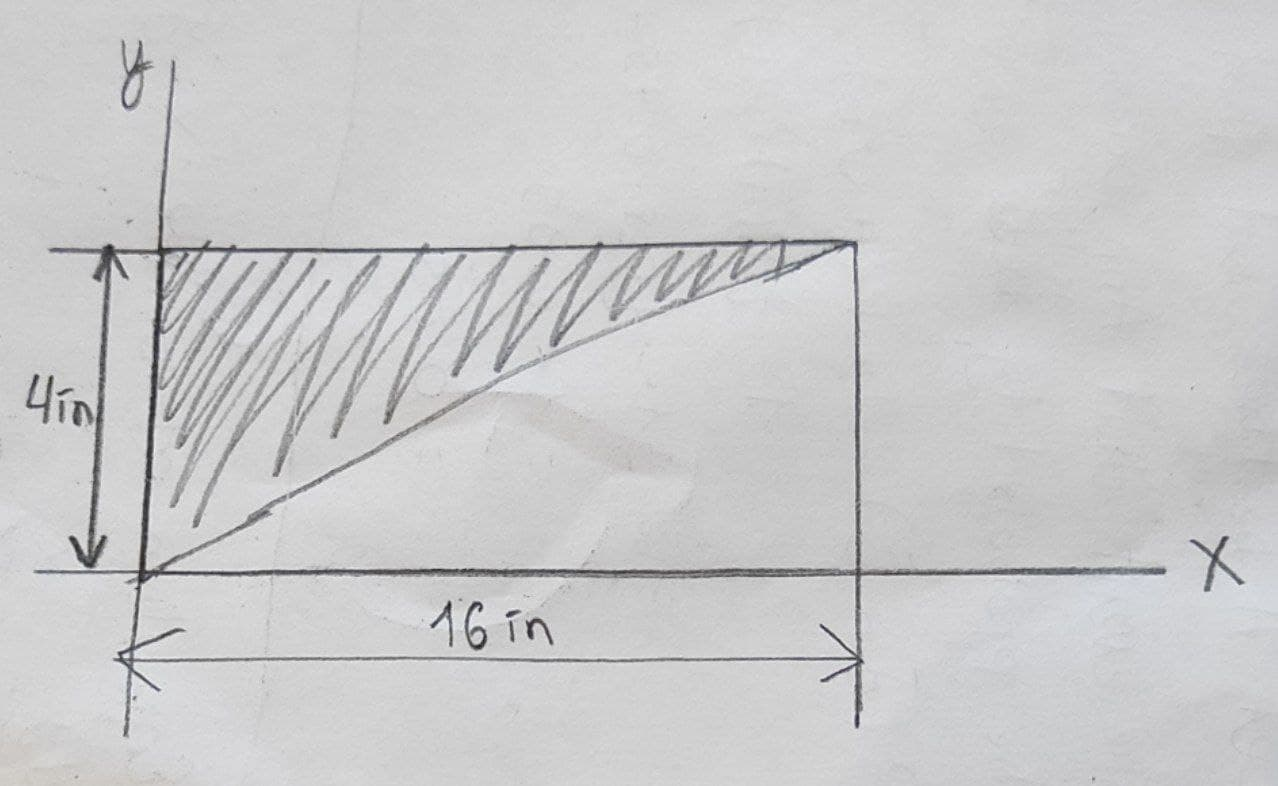Marenonigt

2022-01-14

Determine the moment of inertia for the shaded area about the x axısenlacamig

Write the equation of the shaded area.
${y}^{2}=x$
$x={y}^{2}$
Consider a rectangular differential element with respect to the y-axis with a thickness dy and intersects the boundary at (x, y).
Express the area of the differential element parallel to the x-axis.
$dA=xdy$
Substitute ${y}^{2}$ for x.
$dA={y}^{2}dy$
Calculate the moment of inertia for the shaded area about the x-axis.${I}_{x}={\int }_{A}{y}^{2}dA$
Substitute ${y}^{2}dy$sonorous9n

at the end, should be 1/5 instead of 1/7alenahelenash

Yes, it should be $\frac{{y}^{5}}{5}$